# Hexagon Shape

In geometry, a hexagon is a closed two-dimensional six-sided polygon. It is made up of six line segments and six vertices that form six internal angles. The sum of all the internal angles of a hexagon is 720°. Also, the shape of a hexagon is given in the figure below: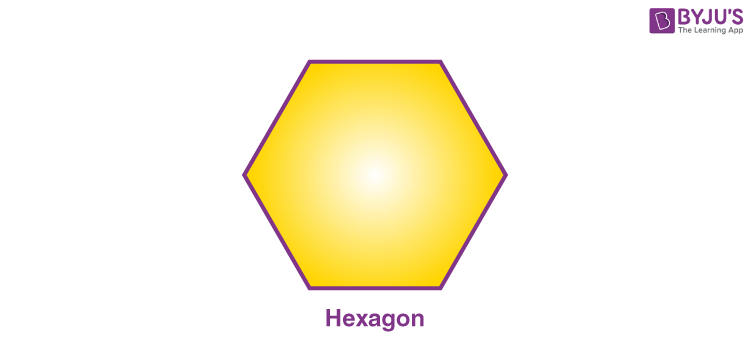## Hexagon Shape Images

We can categorise the hexagonal shape into several types based on the measure of sides and angles. These are explained here with the suitable figures and examples.

Regular Hexagon

A hexagon is said to be a regular hexagon if the length of all the sides is equal and the measures of all the interior angles are the same. Also, the internal angle is equal to 120°. The regular hexagon consists of six symmetrical lines and rotational symmetry of order of six.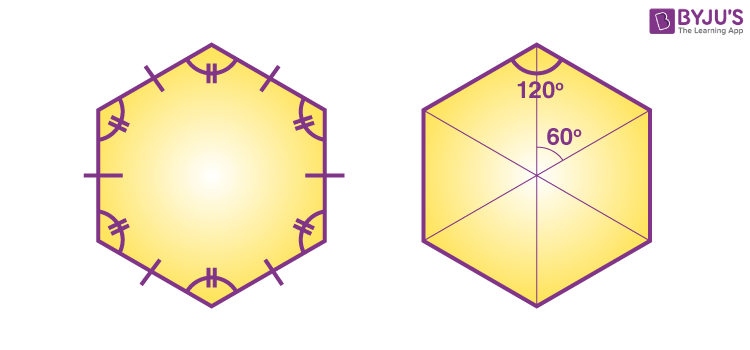Irregular Hexagon

A hexagon with unequal sides and angles is said to be an irregular hexagon. A sample irregular hexagon is shown in the below figure.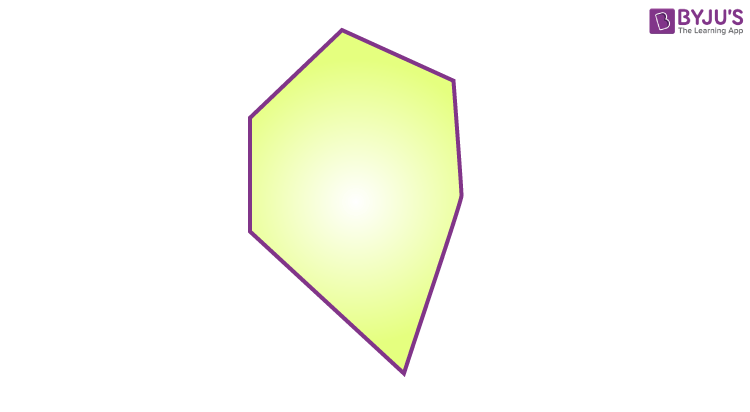Concave hexagon

A hexagon with at least one interior angle greater than 180° is said to be a concave hexagon. In other words, a hexagon with at least one of the vertices points towards inwards. It may be a regular concave or irregular with respect to sides.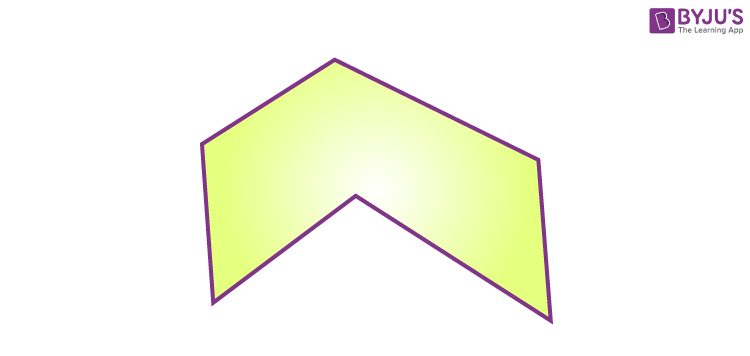Convex hexagon

A convex hexagon is a type of hexagon in which all the interior angles measure less than 180 degrees. Also, it has all the vertices pointing outwards from the center. This is a common type of hexagon that we regularly use when dealing with shapes in geometry. Also, it may be a regular or irregular type of hexagon.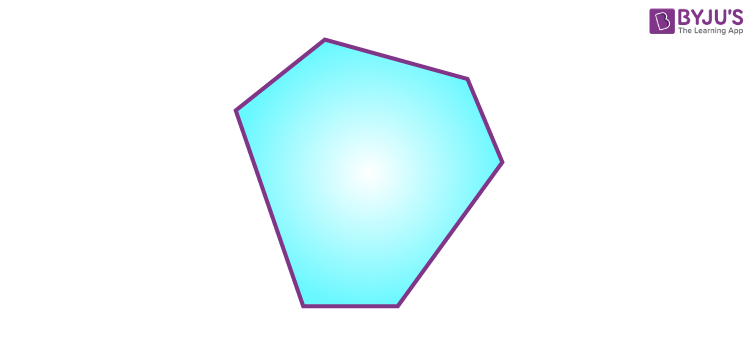The properties of hexagon cover all the parameters such as sides, angles, perimeter, area and so on.

### Hexagon Shape Objects

We may observe different objects that have the shape of a hexagon. A honeycomb, a nut, white divisions of a volleyball, stop board in traffic symbols, back end of pencils and bolts are all examples of real life objects that have the shape of a hexagon. These shapes have been illustrated in the figure below to understand in detail.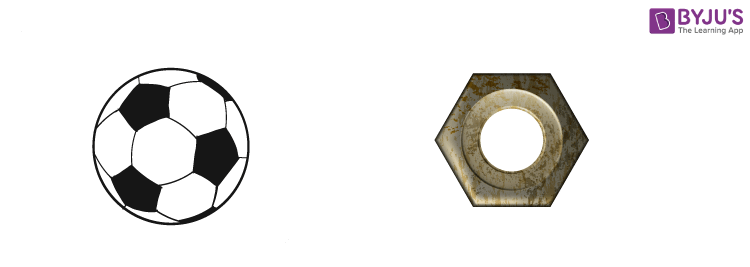As mentioned above, regular hexagonal shapes are generally considered while dealing with various problems in geometry. Let us have a look at the basic parameters of this type of hexagon here.

### Perimeter of a Hexagon

Perimeter of a polygon is the total boundary of the polygon around it. The formula to find the perimeter of a hexagon with all its sides equal is given by:

Perimeter (P) = 6a

Here, a is the length of all congruent sides

If a, b, c, d, e, f are the lengths of sides of an irregular hexagon, then its perimeter is:

P = a + b + c + d + e + f

### Area of Hexagon

The formula to find the area of a hexagon with equal sides is given by:

Area (A) = (Apothem × Perimeter)/2

Here, apothem is the distance between the midpoint of any of the sides and the center of the hexagon.

## Frequently Asked Questions on Hexagon Shape

### Are all 6 sided shapes hexagons?

Yes, all six-sided shapes are called hexagons since a two-dimensional shape or polygon with 6 sides is known as a hexagon. Also, all the sides of a hexagon are straight (i.e. line segments), not curved.

### Are all sides of a hexagon equal?

All the sides of a hexagon are equal only if it is a regular hexagon. By the definition, a regular hexagon is a type of hexagon with all its sides congruent and all the interior angles are of equal measure.

### What is a hexagon 3d shape called?

Prisms and pyramids sometimes appear as a 3d-hexagon. That means, if the cross section is a hexagon, then the prism is called a hexagonal prism. In case of a pyramid, the base will be in the shape of a hexagon, then it is called a hexagonal pyramid.

### What two shapes can make a hexagon?

We can make a hexagon shape by joining two trapezoids of equal measures in terms of bases. By joining these, 6 sides and 6 vertices will be obtained.

### What is a real life example of a hexagon?

Some of the real life examples of a hexagon include honeycomb, a nut, white divisions of a volleyball, stop board in traffic symbols, back end of the pencil, bolt, etc.

### What is the difference between a hexagon and a regular hexagon?

The main difference between a hexagon and a regular hexagon is that a hexagon is a six sided polygon in two-dimensional area, whereas a regular hexagon is a type of hexagon with congruent sides and internal angles.